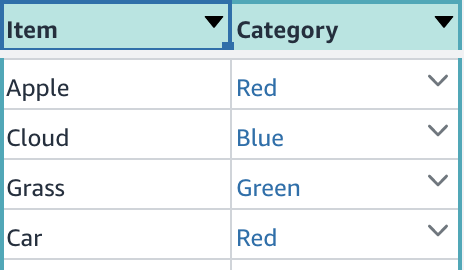# Show weight percentages in a picklist

Hi there,

I wanted to share this in case someone comes across the same problem. I want to see weight percentages (proportion ratios) in a picklist. Consider I have 3 categories in a picklist, each have a count based on how many references it got. So far it's a standard ordinary picklist.

• Red: 4
• Blue: 2
• Green: 4I would like to know the percentage of each item out of the total count, i.e. see their weight out of 100% as follows in the weight column of the picklist:

• Red: 40%
• Blue: 20%
• Green: 40%

In the table used as the picklist, I added a 3rd column called Percentage and used the following formula:
`=ROWS(FILTER(T_Items,"T_Items[Category]=THISROW()"))/ROWS(T_Items)`

Then set the 3rd column's format to Percentage.

You can create a Column List in your app based on this picklist with 3 columns to see a summary report of the number of items and their proportional "weight" percentage.

Thanks,
Andras

3 Likes

Nice example, @AndrasThanks for sharing here for other Honeycoders!